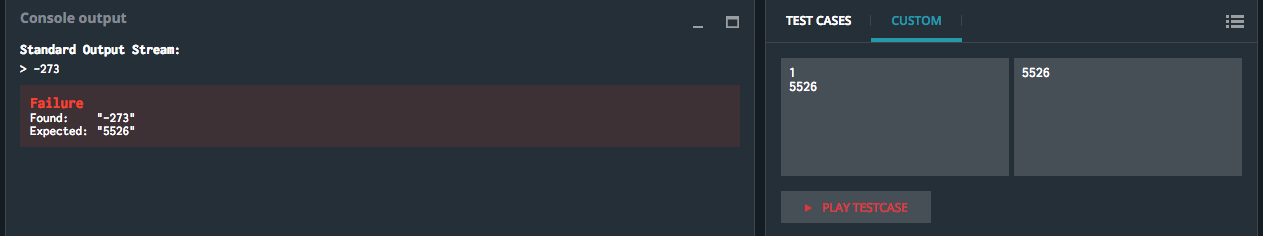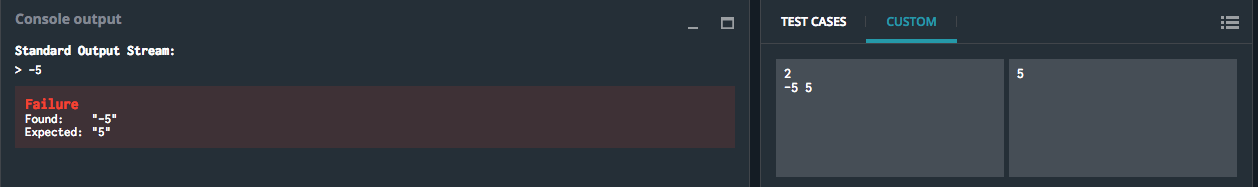# Temperatures puzzle discussion

#648

Nope, you are not stupid.
It’s L as in Line (print and change line).

#649

I’m attempting this in python (still new to programming) can someone help with why this won’t pass the extra validators where there are only positive numbers, but will pass the ones with only negative numbers:

``````if postemp == []:
print(negtemp)
elif negtemp == []:
print(postemp)
``````

To explain, I’ve completed this by passing all negative numbers into negtemp and all postive numbers in to postemp, then sorted them so I get the closest number to 0 in the first position in the list.

What I want to do is if the postemp list is empty, just output the item at position 0 in negtemp, and if the negtemp list is empty output the item in position 0 in postemp. But it still fails the validators with “Simple data set: {7 5 9 1 4}” and “5526 alone”. Everything else passes with no problems, but I can’t get past 72%

Edit:
Figured it out, it was the position of it in my code, due to something I was doing to compare negative numbers against positive. Had to put it before that.

#650

#c++ #cpp

Hello, this is my solution, but something doesn’t work. I checked it few time and all seems good. Could you check it? Maybe you guys can tell me where im wrong. Output:

Standard Output Stream:

5 //negative number from ne
1 // positive one from p
5 // negative from ne
-2 //negative from arrn
-2 //negative from arr

My numbers from  to 
1
-2
-8
4
5
result

Admin edit: don’t share full code pls

#651

working for me, it’s not the best option i think, but works (only 50%). You can give me some ideas to rearrange code or to use other formulas.
admin edit: don’t share full code pls

#652

Hello all,

I’m still quite new to coding, I’m trying to resolve this exercise in Python (3).
However, I think that what is asked in the exercice differs from the tests.

Here is my code.

``````import random

temp_list = list(range(-273,5527))

value = []
n = int(input())
while len(value) < n:
value.append(random.choice(temp_list))

t = ()

if n == 0:
t = n
elif min(value) >= 0:
t = min(value)
else:
value_neg = [e for e in value if e < 0]
value_sup = [e for e in value if e >= 0]
if value_sup == []:
t = max(value_neg)
else:
if max(value_neg)+min(value_sup) > 0:
t = max(value_neg)
elif max(value_neg)+min(value_sup) <= 0:
t = min(value_sup)

print (t)
``````

I don’t answer the 0 <= N < 10 000 condition yet.

For the rest of the conditions, this code takes into account the possibility of having an empty list, that is to say 0 temperatures, the range of possible temperatures starts at -273 and end at 5526. It will choose a positive temperature over a negative one if they have the same absolute value. Finally, it can handle only negative temperatures
To conclude I answer the conditions.

Even though, the test requires to give specific anwsers for the different tests. Why should the answer to “only negative values” be -5? It is asked to have a code that will give you the closest temperatures to 0 not a specific one.

So whether I’m stupid or, the conditions asked and the tests made to validate the code don’t match.

#653

If you take a look at the rules:

Input
Line 1: N, the number of temperatures to analyze
Line 2: The N temperatures expressed as integers ranging from -273 to 5526

You’ll quickly realize that you’re not reading 2nd line of input which contains the actual temperatures that you need to evaluate. Instead of randomly generating your own values you should be reading them from input since they are provided.#654

Where i’ve got mistake, every test i pass but in result i have failure with validator 3. Here’s my code

``````import sys
import math

temperatureplus = 5526
temperatureminus = -273
n = int(input())  # the number of temperatures to analyse
print(n, file=sys.stderr)
for i in input().split():
# t: a temperature expressed as an integer ranging from -273 to 5526
t = int(i)
if t <= temperatureplus and t>=0:
temperatureplus=t
if t >= temperatureminus and t<=0:
temperatureminus=t

# Write an action using print
# To debug: print("Debug messages...", file=sys.stderr)
if n == 0:
print(0)
elif temperatureplus <= abs(temperatureminus):
print(temperatureplus)
else:
print(temperatureminus)``````

#655

For Input [1000, 1200, 600], your answer will be -273 which is incorrect

#656

hey how to solve that +5 and -5 problem…what should be the algo for that??

#657

at least give me any hint
??

#658

can someone please tell what is the error

``````int main()
{
int n;
int t;
scanf("%d", &n);
for (int i = 0; i < n; i++) {
// a temperature expressed as an integer ranging from -273 to 5526
scanf("%d", &t[i]);
}
int min=t;
int min1=t;

for (int i = 0; i < n; i++)
{
if (t[i]>0)

{
if (min>t[i])
{
min=t[i];
}
}
else if (t[i]<=0)
{
if (min1<t[i])
{
min1=t[i];
}
}
}

// Write an action using printf(). DON'T FORGET THE TRAILING \n
// To debug: fprintf(stderr, "Debug messages...\n");

printf("result\n");
if ( (-1*min1)<min)
printf("%d",min1);
else if ( (-1*min1)>min)
printf("%d",min);
else if ( (-1*min1)==min)
printf("%d",min);

return 0;
}
}``````

#659

not sure what i need to do. the preset code given kind of makes sense to me, but i do not know what to add or input to make things work. i am new to python at this level i know very basic syntax and operations. have not written very many full programs/scripts/codes. this is as far as i have gotten, and would appreciate any help.

``````import sys
import math

# Auto-generated code below aims at helping you parse
# the standard input according to the problem statement.

n = int(input())  # the number of temperatures to analyse
for i in input().split():

t = int(i)

# Write an action using print
# To debug: print("Debug messages...", file=sys.stderr)

print(i)``````

#660

For each new ‘t’, you will need to find if it’s closer to zero than the previous value.

• You will have to find a way to store the ‘closest to zero I have seen so far’ value
• Compare this ‘closest’ value to ‘t’ in each iteration and update ‘closest’ with ‘t’ if it’s relevant
• You can look the function ‘abs’ to be able to compare negative and positive value

With that you should at least be able to pass the first 2 steps

#661

Help, this code works with all the testcases except for 4, can some one tell my why.
Its in JavaScript.

``````   /**
* Auto-generated code below aims at helping you parse
* the standard input according to the problem statement.
**/

var n = parseInt(readline()); // the number of temperatures to analyse
var inputs = readline().split(' ');
for (var i = 0; i < n; i++) {
var t = parseInt(inputs[i]); // a temperature expressed as an integer ranging from -273 to 5526
}
// Write an action using print()
// To debug: printErr('Debug messages...');
//print(inputs);
var a = 0;
var b = 0;
var c = 5526;
for (var i = 0; i < n; i++){
if(inputs[i] < 0){
a = inputs[i] * -1;
}else{
a = inputs[i];
}
if(a < c){
c = a;b = inputs[i];
}else if (a == c && b < 0) {
b = inputs[i];
}
}
print(b);``````

#662

here there is a for loop given (using c++)
i thought the values are stored in an array dynamically but there isnt any array initialised. so where are the values stored and how can i access each value individually . please help
thanks

#663

Did you already solved it?

#664

Hi there.I have an issue with temperature task: I have mantioned every condition in my program and it read every thing in a correct way but there is some bug or something which prevents me from completing the quest for 100% It is related to the range where it says to have range from 5526 to -273. It reads my -273 but when it comes to 5526 it is showing that there is nothing like that. PLEASE HELP!!!

#665

same problem as above. JavaScript btw. Also while I completed all the tasks in the execution part with no hard coding and completed tasks such as differing 5 from -5 , but during the validation test, it does not differ the 7 from -7. I don’t know why this happens. Maybe it is a bug or maybe its just the code being wrong. Any suggestion for optimization is appreciated. Here is the code

``````var n = parseInt(readline()); // the number of temperatures to analyse
var inputs = readline().split(' ');

function SayHello(x){
let closest = 0
let integ = 0
integ = x.length
let gg = 

for(i=0;i<integ;i++){
if(x[i] in inputs === true){if((0-gg)<(0-x[i])){
for(let i=0;i<gg.length;i++){
gg.pop()}
gg.push(arr[i])}
else if(0-gg===0-x[i]){
gg.push(arr[i])}
}else{if((0-gg)<(0-x[i])){
for(let i=0;i<gg.length;i++){
gg.pop()}
gg.push(arr[i])}

else if(0-gg===0-x[i]){
gg.push(arr[i])}

}}

closest = gg
if(closest in inputs === true||gg===true){
print(closest)}
else{print(closest*-1)}

}
arr = []

for (var i = 0; i < n; i++) {
var t = parseInt(inputs[i]);
if(t>0){
arr.push(t)}
else{
arr.push(t*-1)}
// a temperature expressed as an integer ranging from -273 to 5526
}

if(arr.length>0){
SayHello(arr)}
else{print(0)}

// Write an action using print()
// To debug: printErr('Debug messages...');`
``````

Yeah that’s the code. Please do help so I can get this puzzle done and over with. Thanks!

#666

this should help @Boolmachine@InCogBug in your code (as 99,9% of the times)

#667

My code seems to be bugged. I am only getting 54% in the validator and I cant seem to return positive 5126. Please do help in resolving my problems so that I can learn from that. All help is appreciated.
Link to the code on hastebin. Temperatures.